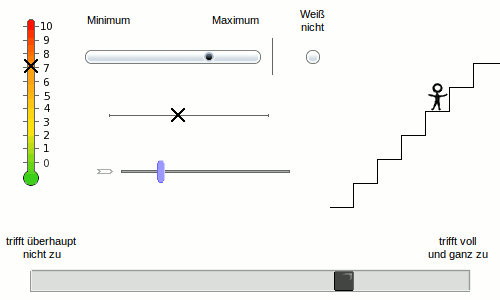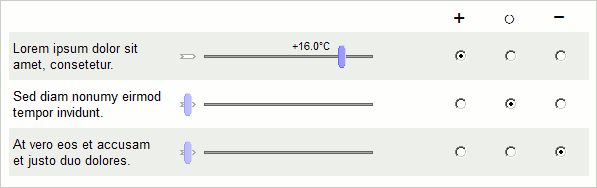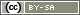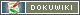[[Slider]]

# Slider

This question type is based on the slider control on a mixing console: the participant can move a button within a defined range. Both the range and the button can be freely customized. This is what makes the slider a multifacted question type – it allows the participant to use visually appealing scale formats, sophisticated setting measurements (see VAS below), a thermometer or a ladder to indicate their level of preference.The slider can be used as a continuous scale (normally a range of 1 to 101) or a certain number of levels can be specified.

Note: Sliders are displayed in the questionnaire using JavaScript. If the participant has deactivated JavaScript, the programme shows an alternative display with 11 selection fields – if a lower differentiation was specified in the question, fewer fields will be shown.

## Visual Analogue Scale (VAS)

You can find a special form of slider in the question templates: the visual analogue scale. This type of slider is made up of a plain line and respondents indicate their answer by moving the mouse pointer to the desired position on the slider.

As a rule, analogue scales provide more accurate data than the classic 5 or 7-point scales. They are also well-suited for bringing diversification into the questionnaire. However, the response time for each item is twice as long as when a 5-point scale is used.

## User-Defined Slider

Each slider consists of two components: a scale (the background) and the slider button (the part that moves). For both components, there is a range of graphics ready to choose from, which can be selected in Graphic for scale and Graphic for slider button.

If you use the predefined scales, as a rule, you do not have to worry where to place the slider button. This will be automatically adjusted.

There is also the option to use your own graphics as a scale and/or slider button:

• Save the respective graphics in a format suitable for the internet (PNG, see Using Pictures).
• Make sure the graphics have the correct pixel dimensions. For example, if a slider should fill the total width of a questionnaire with a width of 600 pixels, then the graphic for the scale must also be 600 pixels wide — maybe even a little narrower so that the labels have space.
• A scale can have room for a “don't know” option, but this is not essential.
• You can also insert text/labels in the scales. However, preferably, you should label the minimum, maximum and “don't know” options in the tab Anchors so that the questionnaire remains accessible (normally text in graphics cannot be read by a screen reader)
• Following this, load the file(s) in Image and Media Files in your project overview. Note down the file names (pay attention to upper/lower case!).
• In a “Slider” question type you can now select the option “use own scale graphic” in Graphic for scale and enter the file name of the uploaded file to the right. After saving, SoSci Survey should set the Scale metrics (i.e. the size in pixels) automatically. The same goes for the slider button.
• After this, you still have to define the correct position of the slider button. To do so, specify where the slider button should be located at minimum position, maximum position and – if provided in the scale – where the “don't know” option can be selected.
• The slider button can move horizontally, vertically or diagonally – depending on where minimum and maximum are located.
• Previewing the questionnaire (directly beneath the metrics) helps to find the correct positioning. Here, the slider button is shown in all three positions at once. If necessary, the correct values have to be determined by trial and error.
• The width of the three positions controls the labeling. This determines how much space the label placed above the scale is given – provided that a label is given in the tab Anchors.
• If the slider should not allow a continuous movement between minimum and maximum, but rather only for certain levels, enter the respective value (max. 20 for discrete steps) for the Differentiation.
• After saving, you can test out the slider in the Preview of the question below straightaway.

## JavaScript Reference

The slider feature is powered by the JavaScript Class `SoSciSliders`. The following methods are available. They are explained in examples below.

• `Slider SoSciSliders.getSlider(String sliderID)`
Identify an slider by it's item ID.
• `void SoSciSliders.setFormat(Function func, [String sliderID])`
Set a function to define which values a slider shall display.
• `void SoSciSliders.setDisplay(Function func, [String sliderID])`
Set a function to define at which position to display a value.

A single slider as returned by `SoSciSliders.getSlider()` is of class `Slider`. This class has the following attributes and methods:

• `int Slider.value`
The current value of the slider (of only one button is set for the slider).
• `boolean Slider.disabled`
Disable the slider.
• `number Slider.getValue(int index, Boolean exakt)`
Return the current value of the slider (if multiple items are used per slider). The paramtere exakt defines if an exact floating point value for the position shall be returned or a rounde value as it will be stored in the data set.
• `void Slider.setValue(number value, int index)`
Set the slider's value (if multiple items are used per slider).
• * `void Slider.fixValue(number value, int index)`
Set the knob of the slider with the index (0 or 1) to the specified value. This locks the knob.
• `object Slider.getButtonPosition(int index)`
The position (center) of the slider specified by index in pixels, relative to the scale. The return value has the properties `x` and `y`.
• `void Slider.addEventListener(string EventID, callable Function)`
Call the function Function when the respondent clicks the slider and (thereby) sets or changes its value. The parameter EventID muss be “click”.

For example, the following HTML/JavaScript code (JavaScript in the Questionnaire) will forward the participant to the next page directly after clicking on the first item of the slider question “AB01” if a valid value has been selected.

```<script type="text/javascript">
<!--
// The slider will not be available before the page has been loaded
var slider = SoSciSliders.getSlider("AB01_01");
// The "click" event will fire when the respondents moves the slider
if (slider.getValue() > 0) {
SoSciTools.submitPage();
}
});
});
</script>```

## Display the Current Position

A slider may display the current position (in percent) above, below, or beside the slider button. Use the option Display value to enable this feature.

Using a bit JavaScript, you may display something different than the percent value. First, you need a function to convert the internal value into the text to display.

Note: Enable the option Display value to show any text next to the slider button at all.

Note: The internal value is between 1 and the Differentiation defined for the slider (default 101).

Note: When the slider is operated, a decimal number is used internally, since the slider moves continuously if a differentiation greater than 20 is set. For output, this value (`value`) may have to be rounded to an integer to get the same value as it will later be stored in the data set: `Math.round(value)`

### Basis-Script

The following JavaScript code displays the set value as it is stored in the data set, i.e. without a percent sign and starting at 1.

```<script type="text/javascript">
<!--
var formatter = function(value, reversed) {
// Display nothing if there is a value less than 0 (e.g. -9 = no response).
if (value < 0) {
return "";

}
// Round the value and return it as texts for display.
return String(Math.round(value));

}

// Use the function defined above for all sliders on the page.
SoSciSliders.setFormat(formatter);
// -->
</script>```

### JavaScript code integration

• Most comfortable is the input field JavaScript-Code under Further Settingsadditional code directly in the slider question. There the surrounding `<script>` is left out and with `s2["%q.id%"].setFormat(formatter);` you can bind the function directly to the sliders of the individual question.
• You may save the code as text element and place it on the questionnaire page, or
• you may write it into an element HTML code that is placed on the page. The code must be placed below the question.

If you do not want all sliders on the page changed (in the same way), you may specify the slider in the second parameter of the JavaScript function `SoSciSliders.setFormat()`:

```SoSciSliders.setFormat(formatter, "AB01_02");
SoSciSliders.setFormat(formatter, "AB01_03");```

Note: If you use the JavaScript code above and do not specify the items individually, the formatting function is then applied to all simultaneously visible sliders, not only to those of the respective page. The print preview of the questionnaire (proof and completed questionnaires) may therefore be incorrect.

Tip: The name `formatter` used for the formatting function was chosen arbitrarily. You may define different functions to display different values in different sliders.

### Example: Euro Amounts

The following HTML/JavaScript code transforms the value range from 1-101 to 0-20, rounds it to whole numbers, and displays a Euro symbol after the value.

```var formatter = function(value, reversed) {
// Don't display anything if a value is less than 0 (e.g., -9 = no response)
if (value < 0) return "";
// Transform the value from 1-101 to 0-100 (subtracting 1) and round using Math.round(),
// then to the range 0-1 (divided by 100),
// then to the range 0-20 (multiplied by 20), and
// finally, add a space and the Euro symbol (plus " €")
return String(Math.round((value - 1) / 100 * 20)) + " €";
}
SoSciSliders.setFormat(formatter);```

### Example: Negative values

This JavaScript code (it has to be places within a `<script>` tag as the code above) displays a number between -25.0°C and +25.0°C. The JavaScript method `toFixed()` takes care of the decimal place.

```var formatter = function(value) {
if (value < 0) return "";
var text = ((value - 51) / 50 * 25).toFixed(1) + "°C";
if (value > 51) {
text = "+" + text;
}
return text;
}
SoSciSliders.setFormat(formatter);```

### Example: Time

In order to display a time value between “0:00 hours” and “24:00 hours” with a step size of 15 minutes, a differentiation “1..97” must be set in the slider (4 steps times 24 hours plus the value 24:00).

```<script type="text/javascript">
<!--
var formatter = function(value, reversed) {
if (value < 0) return "";
// Transformation to 0..96
value = Math.round(value - 1);
// Determine hours and minutes by division/rest (modulo) with 4
var hours = Math.floor(value / 4);
var minutes = Math.floor(60 * (value % 4) / 4);
// Always display the minutes in two digits
if (minutes < 10) minutes = "0" + minutes;
// Connect the values with a colon
return hours + ":" + minutes + " hours";
}
SoSciSliders.setFormat(formatter);
// -->
</script>```

With the same code you can of course request a time, you just have to exchange the “ hour ” at the end against “clock”.

### Example: Percentages

In this example, a percentage distribution, e.g. “40% / 60%” is to be displayed. A differentiation “1..101” must be set in the slider (101 steps from 0 to 100%).

```<script type="text/javascript">
<!--
var formatter = function(value, reversed) {
if (value < 0) return "";
// The percentage value is the internal value minus 1
var percent = Math.round(value - 1);
return percent + "%&nbsp;/&nbsp;" + (100 - percent) + "%";
}
SoSciSliders.setFormat(formatter);
SoSciSliders.setDisplay("top");
// -->
</script>```

The `&nbsp;` is a protected blank space. Alternatively, you can use a positioning function (see below) which increases the width of the display field a little.

### Example: Display for individual sliders

Using JavaScript, displaying of the position may also be disabled for single sliders. This HTML/JavaScript code disables the display for the second slider of question “AB01”.

```<script type="text/javascript">
<!--
SoSciSliders.setDisplay(null, "AB01_02");
// -->
</script>```

### Example Positioning of the displayed value

Maybe, you need to adjust the position of the displayed value. To do so, you need to define a function returning the desired position of the label (center `x`, `y`) and optional its with (`width` in pixels), its alignment (`align`) and fontsize (`fontSize`) as object. This function receives 6 parameters: The position of the slider button (center x, y), the relative position on the scale (range 0 to 1), the label's width and height, and the index of the slider button – in case a secondary button is enabled.

This JavaScript code (it need to be nested within a `<script>` tag, see above) displays labels in slider “AB01_01”, placing the label of the first button on the upper left of this button, and the second button's label on its upper right.

```function positioner(x, y, position, width, height, buttonIndex) {
if (button == 0) {
return {
x:x - width / 2,
y:y - 20,
align:"right"
}
} else {
return {
x:x + width / 2,
y:y - 20,
align:"left"
}
}
}

SoSciSliders.setDisplay(positioner, "AB01_01");```

## Keyboard Input

You may want to connect a text input (e.g., from the question types “Text Inputs” or “Cloze Text”) with the slider.

If the slider (e.g., “SR01_01”) and the text input (e.g., “TE01_01”) are placed on the same questionnaire page, add a text element with the subsequent content (formatting: “HTML code”):

```<script type="text/javascript">
<!--

SoSciSliders.getSlider("SR01_01").attachInput("TE01_01");
});

// -->
</script>```

Advice: The JavaScript function `attachInput()` expects the HTML ID of the text input as parameter.

Tip: You can place the text input anywhere on the questionnaire page: Placing input fields freely

## Attach a don't know option

The templates for slider questions (to be found at New Question) already provides sliders with a “don't know” (DK) option. Yet, they support only one-sided sliders, and only one DK option per slider.

This example shows how to employ multiple DK options per slider. To do so, a slider is combined with a fully-labelled scale (Combined Questions). For the example, the slider has the ID “AB01” and the scale, containing 3 options, has the ID “AB02”. While the first option (code 1) indicates a selection in the slider, the other both options (codes 2 and 3) shall disable the slider.

Tip: If you prefer not to display a third option for the active slider, please go to Compose Questionnaire → tab Settings and enable the option that allows respondents to se-select selected options.This PHP and HTML/JavaScript code is required for the combined question.

`question('AB01', 'combine=AB02');`

The JavaScript code shall be stored as text element. Important: Before the JavaScript the HTML code `<script type="text/javascript">` is required, and below `</script>`, to embed the JavaScript in HTML.

```// Define questions
var sliderID = "AB01";
var scaleID = "AB02";
// Define items to tune
var items = [1,2,3];
// Define scale options to disable the slider
var scaleDK = [2,3];
// Define scale options to enable the slider
var scaleVA = ;
// Remember slider values before disabling
var sliderValues = []

// Convenience function
function makeID(questionID, item, option) {
var itemID = String(item);
if (itemID.length < 2) {
itemID = "0" + itemID;
}
var itemFull = questionID + "_" + itemID;
if (!option) {
return itemFull;
}
return itemFull + option;
}

// Function to care about setting the correct values
function refreshSlider(item, status) {
var slider = SoSciSliders.getSlider(makeID(sliderID, item));
// Optionally reset slider value
if (status) {
if ((slider.value == -9) && sliderValues[item]) {
slider.value = sliderValues[item];
}
} else {
sliderValues[item] = slider.value;
slider.value = -9;
}
// Optionally disable slider
slider.disabled = !status;
}

// Function to select the (first) enabled-option if slider is used
function refreshScale(item) {
if (scaleVA.length < 1) {
return;
}
var slider = SoSciSliders.getSlider(makeID(sliderID, item));
if (slider.value > 0) {
var option = scaleVA;
var scaleOption = document.getElementById(makeID(scaleID, item, option));
scaleOption.checked = true;
}
}

// Attach events to the scale

for (var ii=0; ii<items.length; ii++) {
var item = items[ii];
for (var io=0; io<scaleDK.length; io++) {
var option = scaleDK[io];
var scaleOption = document.getElementById(makeID(scaleID, item, option));
// This one employs a closure
SoSciTools.attachEvent(scaleOption, "click", function(item, status) {
return function(evt) {
refreshSlider(item, status);
}
}(item, false));
}
// Same for enabling options
for (var io=0; io<scaleVA.length; io++) {
var option = scaleVA[io];
var scaleOption = document.getElementById(makeID(scaleID, item, option));
// This one employs a closure
SoSciTools.attachEvent(scaleOption, "click", function(item, status) {
return function(evt) {
refreshSlider(item, status);
}
}(item, true));
}
// And also attach event to the sliders
var slider = SoSciSliders.getSlider(makeID(sliderID, item));
return function(evt) {
refreshScale(item);
}
}(item));
}

});```

## Displaying the Sum of Sliders

Displaying the current sum of all sliders requires an HTML element with an ID where you can “write” the value. This can be placed within an element of the question (title, explanation, etc.) or as separate HTML code on the page.

```<div>
The current sum is <span id="output">-</span>%.
</div>```

The JavaScript code must then iterate through all sliders on every change (`"change"`). This is handled by the `refresh()` function. If the value of a slider is greater than 0 (not on the “no response” position), then the value is added to the sum. In the following code, the value is first rounded (sliders can provide non-integer values) and 1 is subtracted because the value range here goes from 1 to 101, but these values represent 0 to 100%.

```window.addEventListener("load", function() {
var slider = s2.SR01;
var items = slider.items;

function refresh() {
var sum = 0;
for (var key in items) {
var value = items[key].value;
if (value > 0) {
sum+= Math.round(value) - 1;
}
}
document.getElementById("output").innerHTML = sum;
}

for (var key in items) {